# Supporting the Standards for Mathematical Practice With Exemplars Performance Tasks and Rubric at the First Grade Level

Written By: Deborah Armitage, M.Ed., Exemplars Math Consultant

##### Summer Blog Series Overview:

Exemplars performance-based material is a supplemental resource that provides teachers with an effective way to implement the Common Core through problem solving. This blog represents Part 2 of a six-part series that features a problem-solving task linked to a CCSS for Mathematical Content and a student’s solution in grades K–5. Evidence of all eight CCSS for Mathematical Practice will be exhibited by the end of the series.

The Exemplars Standards-Based Math Rubric allows teachers to examine student work against a set of analytic criteria that consists of the following categories: Problem Solving, Reasoning and Proof, Communication, Connections and Representation. There are four performance/achievement levels: Novice, Apprentice, Practitioner (meets the standard) and Expert. The Novice and Apprentice levels support a student’s progress toward being able to apply the criteria of a Practitioner and Expert. It is at these higher levels of achievement where support for the Mathematical Practices is found.

Exemplars problem-solving tasks provide students with an opportunity to apply their conceptual understanding of standards, mathematical processes and skills. Observing student anchor papers with assessment rationales that demonstrate the alignment between the Exemplars assessment rubric and the CCSS for Mathematical Content and Mathematical Practice can be insightful for educators. Anchor papers and assessment rationales provide examples of what to look for in your own students’ work. Examples of Exemplars rubric criteria and the Mathematical Practices are embedded in the assessment rationales at the bottom of the page. The full version of our rubric may be accessed here. It is often helpful to have this in hand while reviewing a piece of student work.

#### Blog 2: Observations at the First Grade Level

The second anchor paper and set of assessment rationales we’ll review in this series is taken from a first grade student’s solution for the task, “A Birdbath.” In this piece, you’ll notice that the teacher has “scribed” the student’s oral explanation. This practice was also used with the Kindergarten task that was published in the first blog. Scribing allows teachers to fully capture the mathematical reasoning of early writers.

“A Birdbath” is one of a number of Exemplars tasks aligned to the Operations and Algebraic Thinking Standard 1.OA.6. This task would be used toward the end of the learning time allocated to this standard. “A Birdbath” provides first grade students with an opportunity to apply different strategies to find the sum of addends six and 14 by decomposing six into five and one and decomposing 14 into 10 and four, or by finding the sum of six and four and adding that sum to 10. The student can use counters, ten frames, a Rekenrek, number lines or a tally chart to support her/his numerical thinking.

Leah counts the birds that came to her birdbath. In the morning, Leah counts six birds that came to her birdbath. In the afternoon, Leah counts fourteen birds that came to her birdbath. Leah says nineteen birds came to her birdbath. Is Leah correct? Show all your mathematical thinking.

Common Core Alignments

• Content Standard 1.OA.6: Add and subtract within 20, demonstrating fluency for addition and subtraction within 10. Use strategies such as counting on; making ten (e.g., 8 + 6 = 8 + 2 + 4 = 10 + 4 = 14); decomposing a number leading to a ten (e.g., 13 – 4 = 13 – 3 – 1 = 10 – 1 = 9); using the relationship between addition and subtraction (e.g., knowing that 8 + 4 = 12, one knows 12 – 8 = 4); and creating equivalent but easier or known sums (e.g., adding 6 + 7 by creating the known equivalent 6 + 6 + 1 = 12 + 1 = 13).
• Mathematical Practices: MP1, MP2, MP3, MP4, MP5, MP6, MP7
###### Click on the orange arrow to the right of the solution below to view Exemplars scoring rationale and rubric criteria.
1 / 6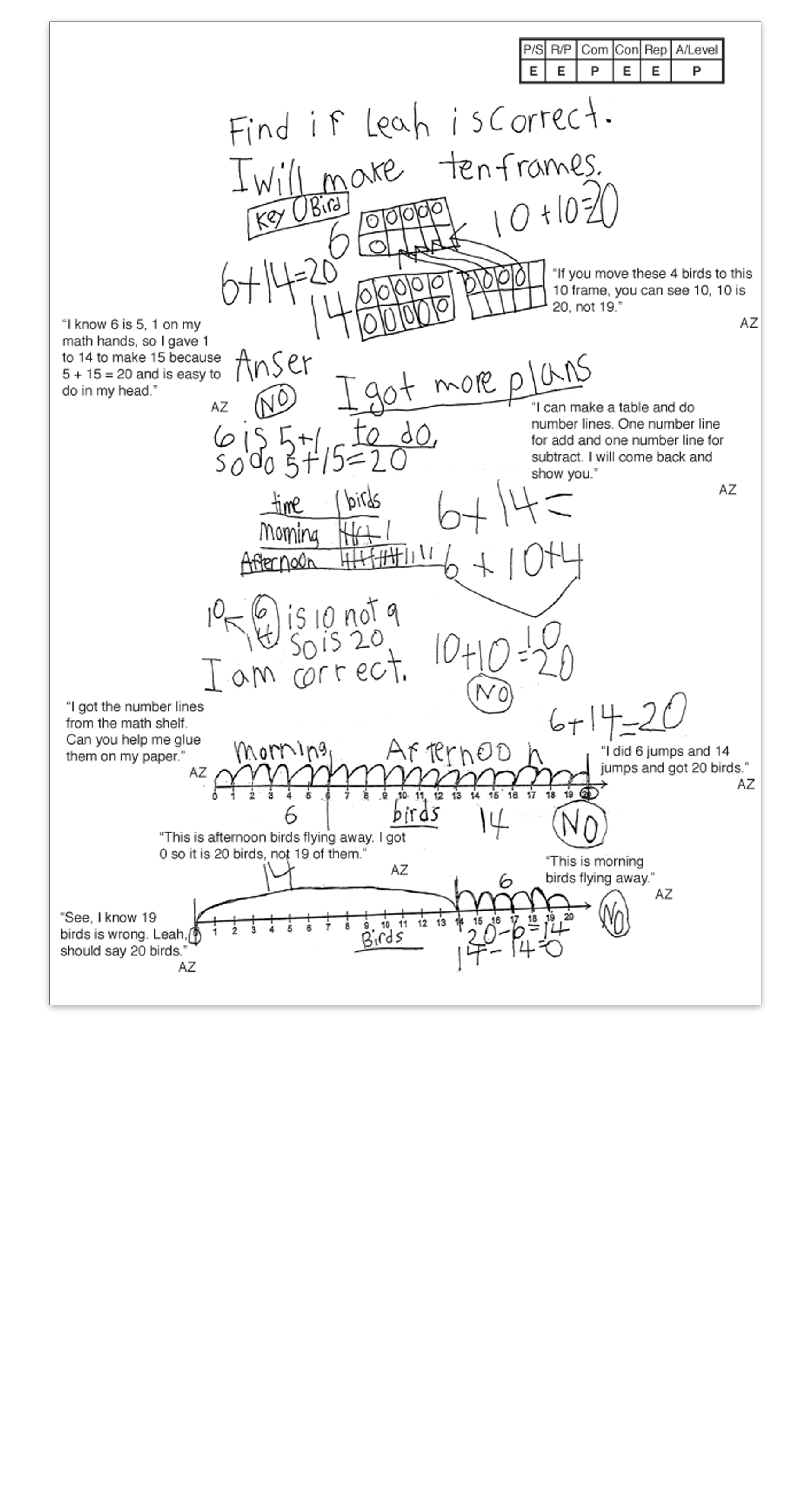2 / 6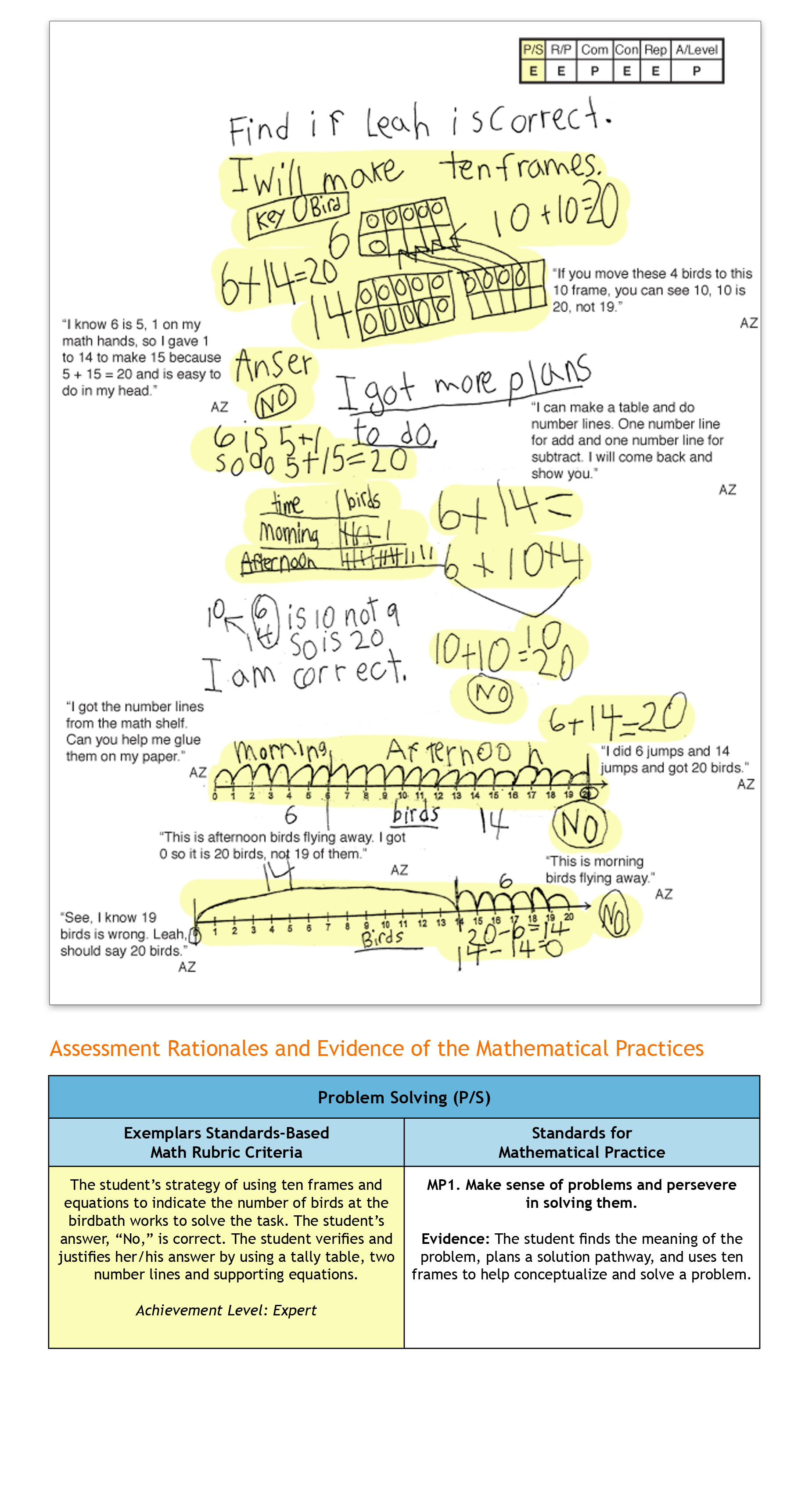3 / 6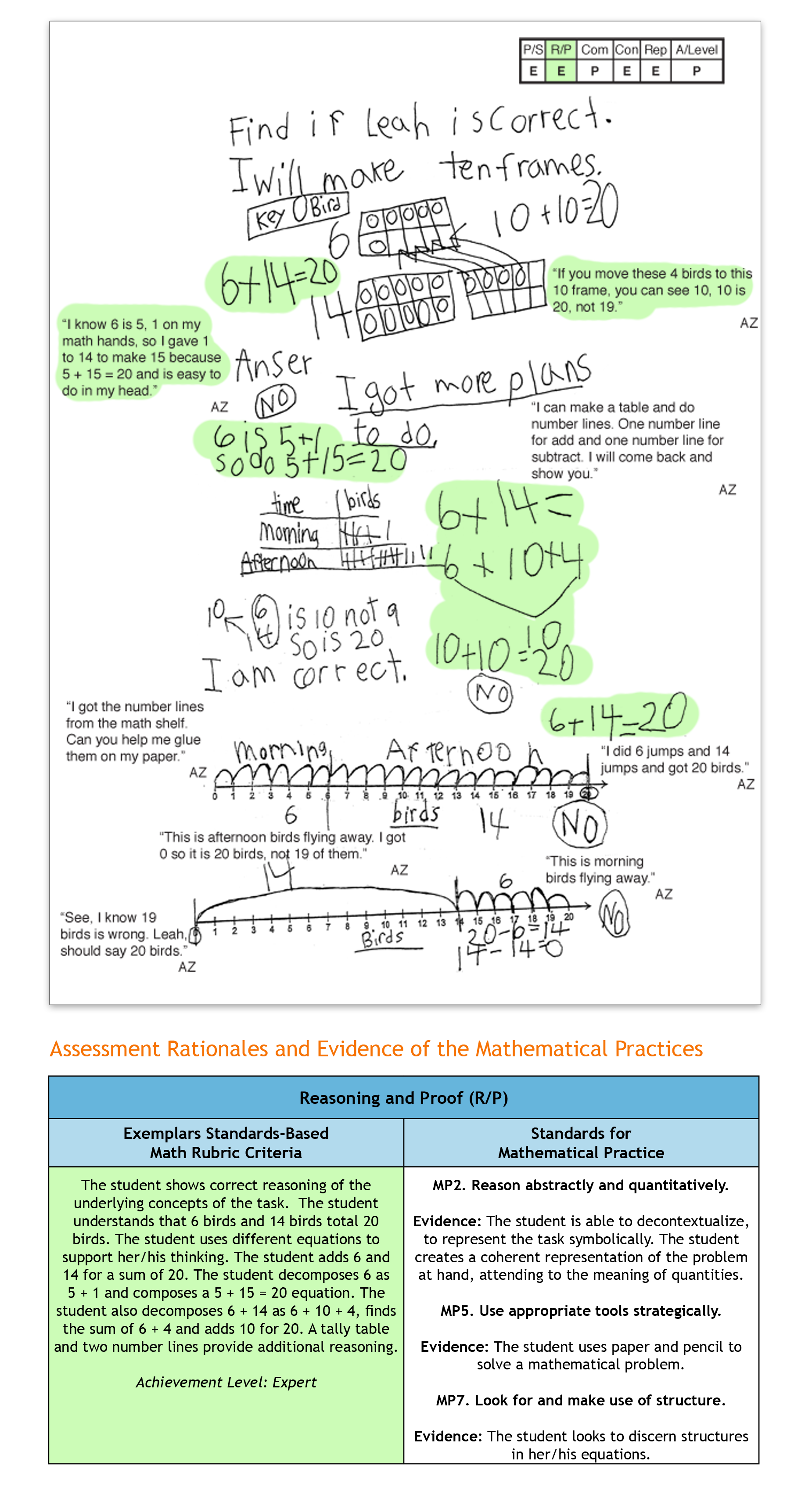4 / 6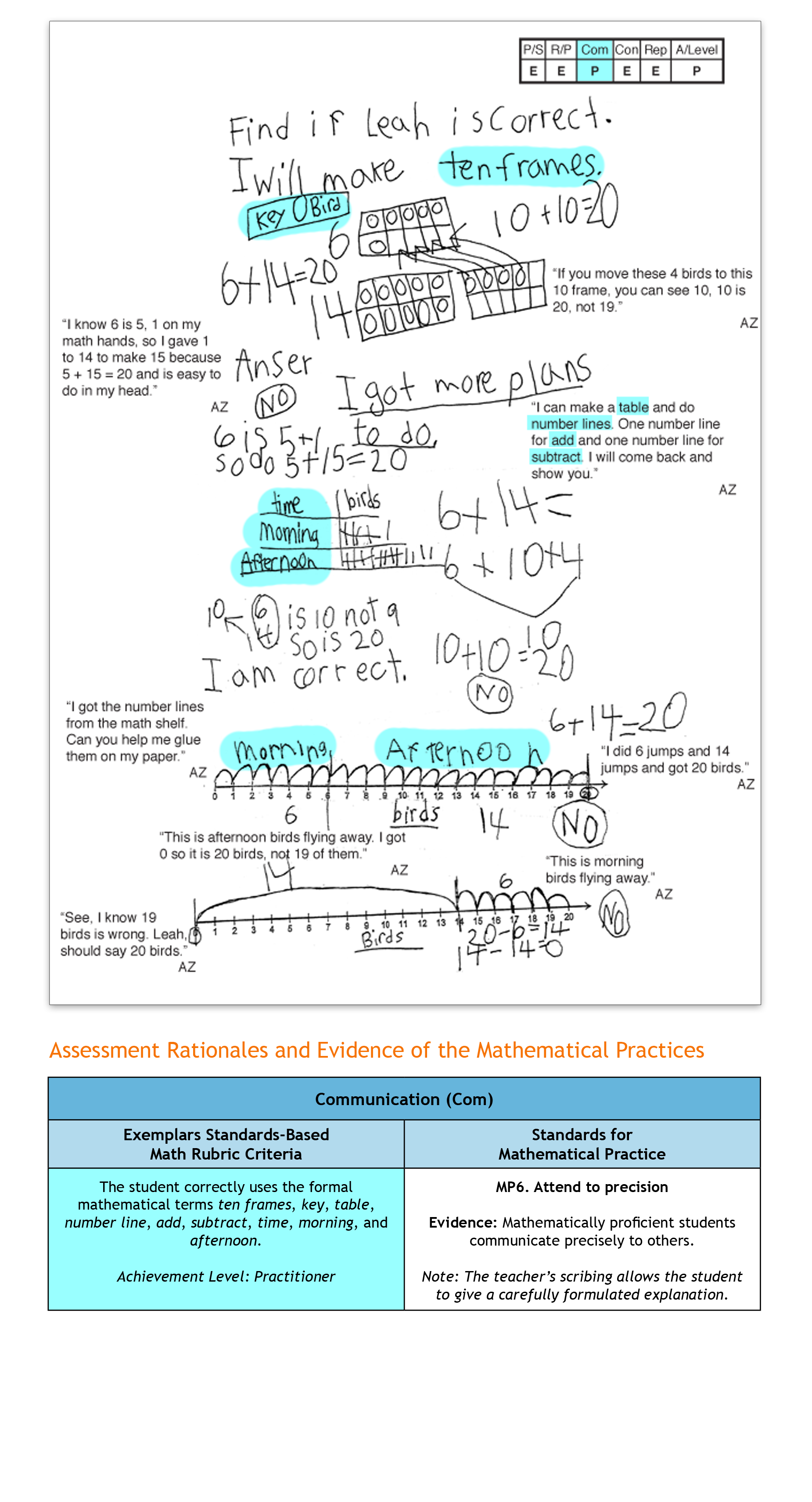5 / 6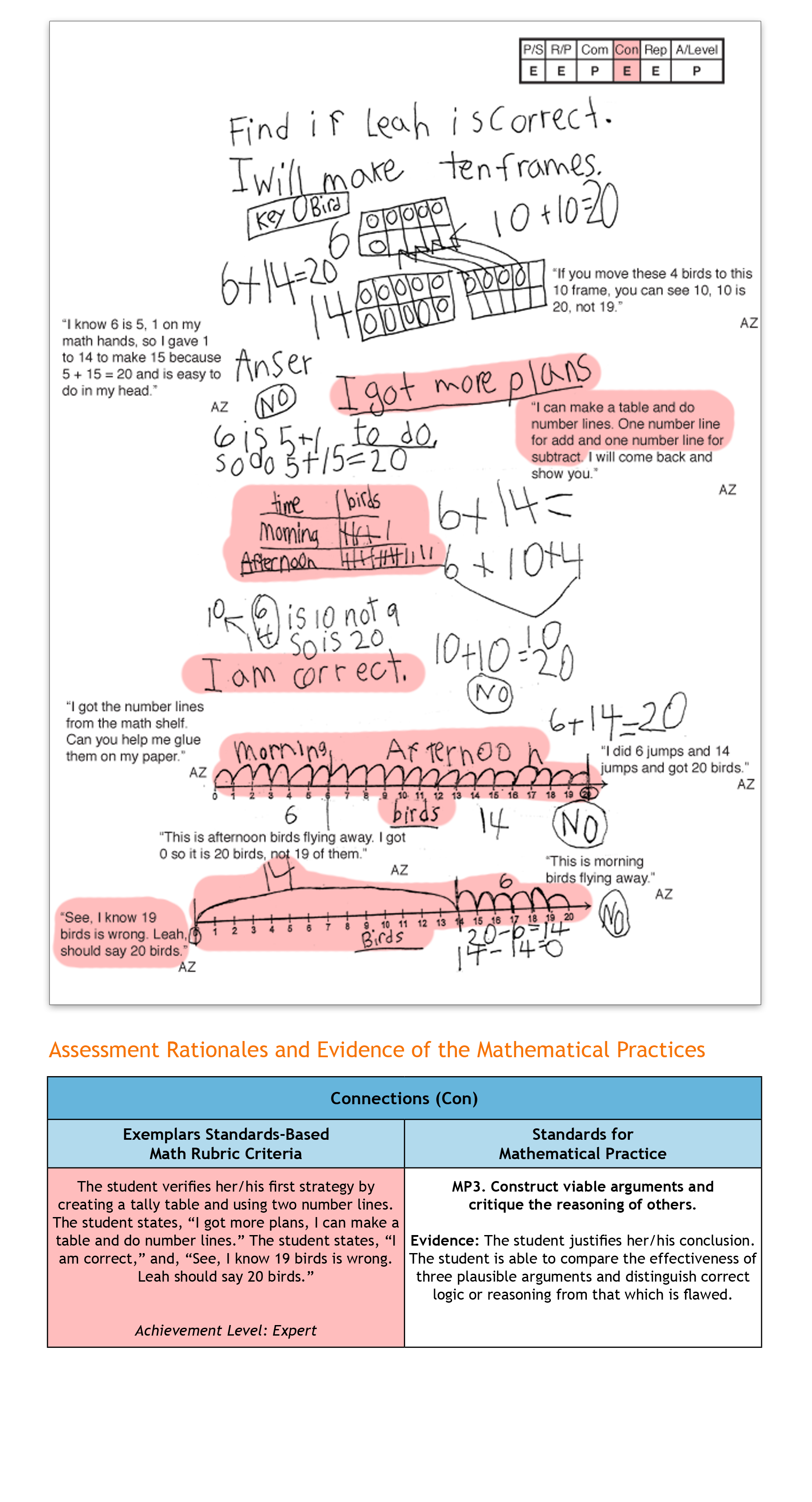6 / 6Topics: